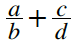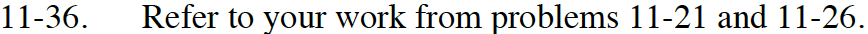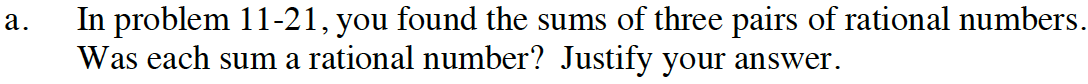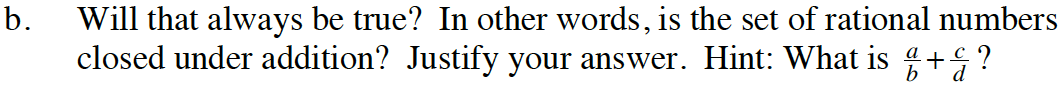Home > INT3 > Chapter 11 > Lesson 11.1.3 > Problem11-36

11-36.
1. Refer to your work from problems 11‑21 and 11‑26. Homework Help ✎

1. In problem 11‑21, you found the sums of three pairs of rational numbers. Was each sum a rational number? Justify your answer.

2. Will that always be true? In other words, is the set of rational numbers closed under addition? Justify your answer. Hint: What is?Yes, each sum was a fraction and therefore a rational number.$\text{Is your answer to }\frac{a}{b}+\frac{c}{d} \text{ another rational number?}$